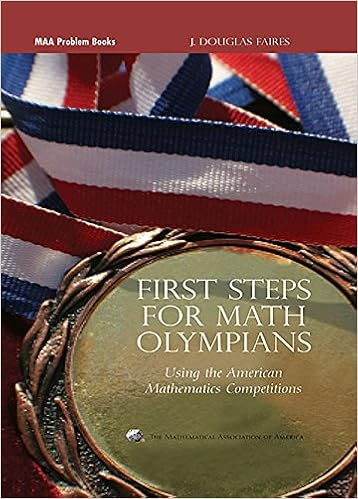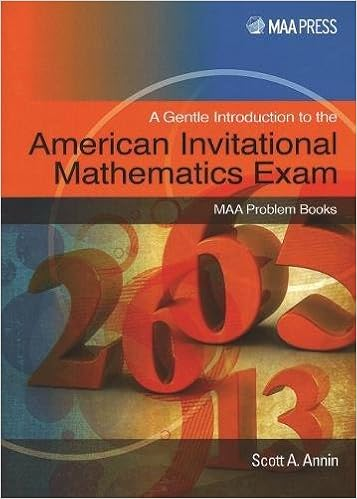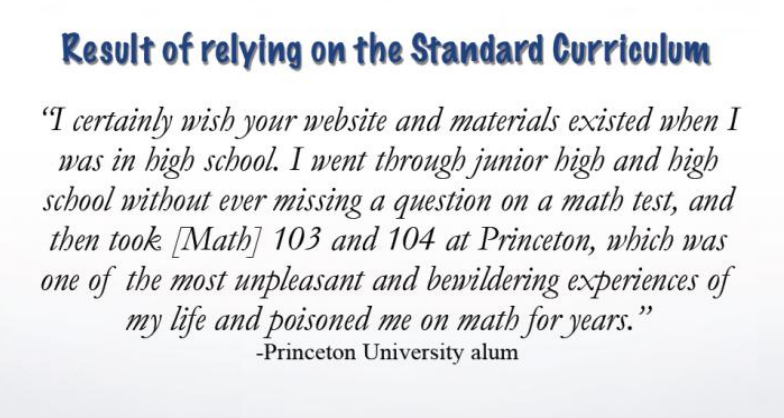# Try it and see if it works

I was tutoring a student who is using the AoPS Prealgebra textbook, and we were learning how to solve linear equations with one variable (p. 215).  This is the sort of problem that looks like this:$21n + 28 = 10n - 40$.
My student understood that in order to solve the equation we first need to combine like terms and isolate the variable, which he successfully did:$11n = -68$.
My student also understood that we need our variable$n$ to have a coefficient of$1$ so that we get something that looks like$n =$ some number.
But how to turn$11n =$ into$n =$?
It turned out he had a lot of interesting ideas.  “How about we subtract$10n$ from both sides?” he suggested.
Those of you well-versed in algebraic manipulations can foretell that this will lead you farther away from a solution.  But rather than cut him off and tell him this is wrong, I went along with it.
“Okay, let’s give it a try and see what happens.  Subtracting$10n$ from both sides…”$11n - 10n = -68 - 10n$
So:$n = -68 - 10n$.
Well, that didn’t work.  It turned out my student had many, many creative approaches to isolating n and solving this problem, none of which got us closer to actually solving the problem.  But rather than cutting him off and prematurely telling him he was wrong, I went along with the playful exploration.  This approach of “try it and see if it works” experimentation is something we want to cultivate in our students, not just in math, but in many academic fields.   Eventually he remembered that we could divide both sides by 11 to solve the problem.   While this sort of discovery approach can be inefficient and tedious, it’s fun to pop into a rabbit hole occasionally.
My hope is that in the future, if he sees another problem like this, he won’t panic, but will instead try to reason it out, just as he did when he learned it for the first time.

# Mental Math Shortcuts

Today my MathCounts team practiced their first Countdown Round.  This is the spelling bee style competition in which students compete head-to-head on stage to answer the question before their opponent.  Identifying elegant shortcuts is critical to getting the answer asap.  For example:(Source: 2016 MathCounts Chapter Countdown Round)

The question asks for the difference between the average time and 10 minutes.  While one could find the average of the 3 times and then subtract from 10 minutes, a more clever approach (using smaller numbers) is to average the 3 differences from the start.  Since$10:13 > 10:00$$t_1 = -13$.  The other time differences are$9$ and$22$.  The sum is$-13+9+22=18$ and the average is$6.$  Smaller numbers means quicker, more accurate calculations.

When I coach my MathCounts teams, I make sure to remind them to answer the question that is being asked.  That is, after all the calculations have been performed and you have a value for x, reread the question, and make sure x is what the problem is asking for.  Only when you are sure you have answered the question should you write it down (or bubble it in).
For example, today I’m reviewing materials for my team, and I came across this problem:
“The three-digit integer$5A4$ is a multiple of six.  What is the sum of all the possible values for the digit represented by A?”
After some calculations, I found 4 possible values for A: 0, 3, 6, and 9.  My first thought was: “The answer is 4!  There are four possible values of A.”  But then I reread the question, and it asks for the sum of all possible values.  The correct answer is$0+3+6+9 = 18.$
Sometimes you can avoid this error by setting  your variable equal to the value the problem is asking for, so that when you do solve for x, you do not need to perform additional calculations to generate the correct answer.
This is a well-known error, and Richard Rusczyk has written about it in the context of developing good problem solving habits.  Some people may describe this as a “trick question” but I think it’s just a matter of taking care to reread the question and answer the question that is being asked, not the question that you wish had been asked.

# Mixed up functions

I recently came across an AIME problem that compared what I call “mixed up functions:”

How many real numbers x satisfy the equation:$\frac{1}{5}(\log_2 x) =sin(5\pi x)$

By mixed up, I mean we have the periodic sine curve equated with the monotonically increasing log function.  Often with logs we can replace them with an exponential function, in this case with base 2, but that looks messy.

The approach I often see with these mis-matched functions is to draw a graph and see where the 2 functions intersect.  But first, I’m going to multiply both sides by 5 because who likes fractions?$\log_2 x =5 sin(5\pi x)$

Ah that’s better.  Notice that the maximum and minimum values of$y=5 sin(5\pi x)$ occur when$x=\frac{1}{10}$ and$x=\frac{-1}{10}$, and the maximum and minimum values are 5 and -5.  And of course this is a periodic function that is defined for all real numbers.$y=\log_2 x$ on the other hand is defined only over positive reals, and since it is always increasing from left to right, at some point the value of the function will exceed 5.  Specifically, this will occur for$x>32$.  Likewise,$y=\log_2 x$ will be less than -5 for$x<\frac{1}{32}$.

And it’s apparent that in the interval$\frac{1}{32} the log function is going to intersect the sine function as it goes up and down on its periodic path.  How many times does it intersect?  AIME writers like to catch students on “off by one” errors, so take care to examine what is happening close to$x=\frac{1}{32}$ and$x=32$ before entering your answer!

# Gentle introductions

The best way to prepare for any test is to locate old versions of the exam and practice with those, especially studying the problems you answered incorrectly.  This is true for the AMCs and MathCounts.  AoPS also offers online prep classes.  For those of you who prefer learning from textbooks, here are  few recommendations.  These books take problems from old competitions and organize them by topic so you can ramp up your skills.Competition Math for Middle School by J. Batterson.  In particular I enjoyed the chapters on counting and probability for their concise and clear introductions to the subjects.  You’ll be up to speed on these topics in no time.  Other chapters are algebra, geometry, and number theory.First Steps for Math Olympians by J. Douglas Faires.  This is the next step in difficulty is preparing for the AMC 10/12.  This book uses questions from those exams as exercises.  Content includes: ratios, polynomials, functions, triangles, circles, polygons, counting, probability, primes, number theory, sequences and series, statistics, trig, 3D geometry, logs, and complex numbers.A Gentle Introduction to the American Invitational Mathematics Exam by Scott Annin.  This title seemed to be speaking to me.  I qualified for AIME my senior year of high school, but bombed the test, so I’ve been living in fear of the AIME every since.  I’m working through the chapter 1 and I’m pleased to find myself actually solving some problems without assistance.  Contents include:  algebra, combinatorics, probability, number theory, sequences/series, logs, trig, complex numbers, polynomials, plane geometry, and 3D geometry.  Nearly all exercises are former AIME problems.

# College Prep

Lately I’ve been finding myself sending a one link to many parents who want to learn more about math education in the US.  So I thought I’d share it here as well.
This is a talk Richard Rusczyk gave at the Math Prize for Girls a few years ago.  It’s very long, but the link should take you to 6:41, and the most interesting slide in the presentation.

Richard Rusczyk Talk

But if you want to save more time, I’ve actually transcribed that portion of the talk, just because I think it’s so important.

“Right after I started Art of Problem Solving I received an email from someone who attended Princeton right around the time I did:”“I want you think for a minute what this student’s middle school and high school teachers thought when he went off to Princeton.  They thought, “We succeeded. He went off to Princeton; we’re awesome.” They never saw this.  I’m sure he didn’t go back to his middle school teachers and say, “Yeah what’s up?!?  You didn’t prepare me for this.”

“So they didn’t get this feedback, and this happens a lot.  I saw this a lot at Princeton, this happens a lot now. Kids go through school, some very good schools, they get perfect scores on everything, and then they come to place like MIT, a place like Princeton, they walk into that first year math class, and they see something they’ve never seen before: problems they don’t know how to solve.  And they completely freak out. And that’s a bad time to have these first experiences. Having to overcome initial failure.”

I think some parents are so focused on getting in to some famous college, that they miss the more important goal of a college prep curriculum:  actually preparing our students for college level work.  The great thing about focusing on building skills, rather than building an impressive resume, is that at whatever college your students ends up, famous or not so famous,  they will be more likely to succeed and excel.

# Have an emotional reaction

I’m a big fan of James Tanton’s approach to solving difficult math problems.  Here are his steps:
Step 2.  Have an emotional reaction to the problem.
Step 4.  Do something.
I love Step 2, and I will often complain out loud in front of my students about the difficulty of a problem, the unfairness of it all.  I think it is fine to acknowledge that a problem is hard, that we hardly understand what is being asked, that we think the writer of the problem should be reincarnated as a fruit fly.
If we acknowledge our feelings of dismay and fear, then we normalize them.  When they become normal, then we can take a deep breath, re-read the problem and take a first step at a solution.  If students think their feelings of fear and dismay are not normal, they’ll begin to think they aren’t good at math.   In reality, many students (even mathy ones) react this way on the first read of a difficult problem.

# Stream of Consciousness

I recently wrote to a homeschooling mom about avoiding an error in math documentation called stream of consciousness math notation.  This is basically the equivalent of a run on sentence or no punctuation.  You can probably understand the gist, but it would be inappropriate for formal writing.  Here’s an example:

Let’s say I have 3 apples and you give me 2 more.  Now I have 5.  Then a third person takes 4 away from me.  Here’s the stream of consciousness version of events:$3 + 2 = 5 - 4 = 1$

Here it is as if the equality is meant to say, “and then what happens is…”  In reality the equality sign indicates that whatever is to the left is the same as whatever is to the right.  A chain of equalities means that everything between the equals signs are all equal to each other.  In the example above, I am writing that$3 + 2 = 1$  This is no big deal with such small numbers because we can all see past the error to what is going on.  But I’m convinced that it leads to muddied thinking as the problems become more complex.

If you see this kind of notation, then gently–preferably with humor–encourage your students to use correct logic.

# Is Fred on the committee?

I was just helping a student with his AoPS homework, when I came across the following related problems:

Eight people, including Fred, are in a club.  They decide to form a 3 person committee.  How many possible committees can be formed?

So we are choosing 3 people out of 8 or${8 \choose 3}$.

How many possible committees include Fred?

Since Fred is taking one committee seat, that means we need to choose 2 more people from the remaining seven, or${7 \choose 2}$.

How many possible committees do not include Fred?

Since we can’t choose Fred, we need to choose 3 members from the remaining 7 or${7 \choose 3}$.

Since the total number of committees is equal to the number of of committees with Fred plus the number of committees without Fred, then we can say${8 \choose 3} = {7 \choose 2} +{7 \choose 3}$.

Generally we call this Pascal’s Rule:${n \choose k} = {n-1 \choose k-1} +{n-1 \choose k}$.

# Law of cosines, without the angleI recently twice on the AMCs came across this problem solving technique for finding side lengths in a triangle with a cevian.  (A cevian is a segment from one vertex of a triangle to the opposite side.)  It uses the law of cosines, but the good news is it may not require an angle measure because it takes advantage of the fact that$cos(180-\alpha)=-cos(\alpha)$.  Note in the diagram that the cevian creates these 2 angles.  By the law cosines$a^2=c^2+e^2-2abcos(\alpha)$ and$b^2=d^2+e^2-2abcos(180-\alpha) = d^2+e^2+2abcos(\alpha)$.  Both equations have the length of the cevian,$e$, and the angle$\alpha$, so you can eliminate a variable and solve.